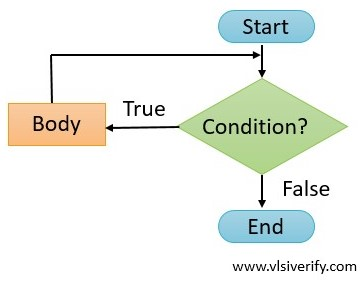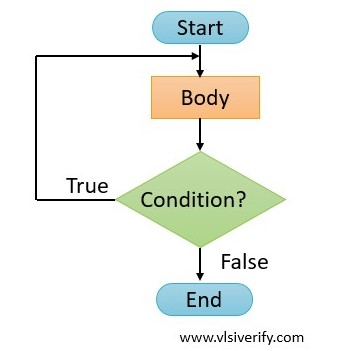Tutorials

A loop is an essential concept of any programming language. The loop is useful to read/ update an array content, execute a few statements multiple times based on a certain condition. In SystemVerilog, we will discuss the following loop blocks.

1. While loop
2. Do while loop
3. Forever loop
4. For loop
5. Foreach loop
6. Repeat loop

In all supported loops, begin and end keywords are used to enclose multiple statements as a single block. A begin and end keywords are optional if the loop encloses a single statement.

## while loop

A while loop is a control flow statement that executes statements repeatedly if the condition holds true else loop terminates.

Syntax:

``````while(<condition>) begin
...
end``````while loop flow chart

### while loop Example

In the below example, the value of ‘count’ incremented till the condition holds true.

``````module while_example;
int count;
initial begin
while(count<10) begin
\$display("Value of count = %0d", count);
count++;
end
end
endmodule``````

Output:

``````Value of count = 0
Value of count = 1
Value of count = 2
Value of count = 3
Value of count = 4
Value of count = 5
Value of count = 6
Value of count = 7
Value of count = 8
Value of count = 9``````

## do while loop

A do while loop is a control flow statement that executes statements at least once and then the condition is checked. If the condition holds true, statements execute repeatedly else the loop terminates.

Syntax:

``````do begin
...
end
while(<condition>);``````do while loop flow chart

### do while loop Example

In the below example, the value of ‘count’ incremented till the condition holds true. But the condition is checked after a loop is executed.

``````module do_while_example;
int count;
initial begin
do begin
\$display("Value of count = %0d", count);
count++;
end
while(count<10);
end
endmodule``````

Output:

``````Value of count = 0
Value of count = 1
Value of count = 2
Value of count = 3
Value of count = 4
Value of count = 5
Value of count = 6
Value of count = 7
Value of count = 8
Value of count = 9``````

## Difference between while and do while loop

In the while loop, a condition is checked first, and if it holds true statements will be executed else the loop terminates.

In do while loop, even if a condition is not true, a loop can execute at once.

Example of do while when a condition is not true:

``````module do_while_example;
int count = 2;
initial begin
do begin
\$display("Value of count = %0d", count);
count++;
end
while(count<1);
end
endmodule``````

Output:

``Value of count = 2``

System Verilog Tutorials